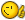# Round down area field in Lee-Mac code

## Recommended PostsPlease, help me in some question. I've been using the lisp the Lee-Mac code for area fields( Areas2FieldV1-3.lsp ) - http://lee-mac.com/areastofield.html, but I need to round down an area values. What should I change in code to get that values?

For example, the value 23,8 m2 should be displayed like 23,5, or 80,23 should be 80,0. Thank you in advance

##### Share on other sitesWelcome to CADTutorUnfortunately, it's not a simple change - replace lines 59-86 (inclusive):

```            (if (= 1 (sslength sel))
(setq str
(strcat
"%<\\AcObjProp Object(%<\\_ObjId "
(LM:ObjectID (vlax-ename->vla-object (ssname sel 0)))
">%).Area \\f \"" fmt "\">%"
)
)
(progn
(repeat (setq idx (sslength sel))
(setq lst
(vl-list*
"%<\\AcObjProp Object(%<\\_ObjId "
(LM:ObjectID (vlax-ename->vla-object (ssname sel (setq idx (1- idx)))))
">%).Area>%" " + "
lst
)
)
)
(setq str
(strcat
"%<\\AcExpr "
(apply 'strcat (reverse (cdr (reverse lst))))
" \\f \"" fmt "\">%"
)
)
)
)```

With the following code:

```            (repeat (setq idx (sslength sel))
(setq lst
(vl-list*
"%<\\AcObjProp Object(%<\\_ObjId "
(LM:ObjectID (vlax-ename->vla-object (ssname sel (setq idx (1- idx)))))
">%).Area>%" " + "
lst
)
)
)
(setq str
(strcat
"%<\\AcExpr (Trunc(("
(apply 'strcat (reverse (cdr (reverse lst))))
")/0.5)*0.5) \\f \"" fmt "\">%"
)
)```

Edited by Lee Mac
•1

##### Share on other sitesThank you very much, Lee Mac! Your code helped me a lot

##### Share on other sitesYou're welcome! @Cadburg##### Share on other sitesOn 12/2/2018 at 8:01 AM, Lee Mac said:

Welcome to CADTutorUnfortunately, it's not a simple change - replace lines 59-86 (inclusive):

```
(if (= 1 (sslength sel))
(setq str
(strcat
"%<\\AcObjProp Object(%<\\_ObjId "
(LM:ObjectID (vlax-ename->vla-object (ssname sel 0)))
">%).Area \\f \"" fmt "\">%"
)
)
(progn
(repeat (setq idx (sslength sel))
(setq lst
(vl-list*
"%<\\AcObjProp Object(%<\\_ObjId "
(LM:ObjectID (vlax-ename->vla-object (ssname sel (setq idx (1- idx)))))
">%).Area>%" " + "
lst
)
)
)
(setq str
(strcat
"%<\\AcExpr "
(apply 'strcat (reverse (cdr (reverse lst))))
" \\f \"" fmt "\">%"
)
)
)
)```

With the following code:

```
(repeat (setq idx (sslength sel))
(setq lst
(vl-list*
"%<\\AcObjProp Object(%<\\_ObjId "
(LM:ObjectID (vlax-ename->vla-object (ssname sel (setq idx (1- idx)))))
">%).Area>%" " + "
lst
)
)
)
(setq str
(strcat
"%<\\AcExpr (Trunc(("
(apply 'strcat (reverse (cdr (reverse lst))))
")/0.5)*0.5) \\f \"" fmt "\">%"
)
)```

Help:

Please, help me in some question. I've been using the lisp the Lee-Mac code for area fields( QuickFieldV1-3 ), but I need to round 5 . What should I change in code to get that values?

For example, the value 11203 should be displayed like 11205, or 11208 should be 11210. Thank you in advance

##### Share on other sitesOn 12/2/2018 at 5:53 PM, Lee Mac said:

You're welcome! @Cadburg;//////////////////////

(defun c:ts1 ( )(LM:QuickField "Length" "%lu2%pr0%ps[,]%ct8" 1))

;//////////////////////////////////////////

(setq str (LM:quickfield:constructfieldstring prop format))

;//////////////////////////////////////////////

(setq Z (/ str 5) )

(setq Z1 (fix Z) )

(setq Z2 (- Z Z1) )

(setq Z3 (IF (>= Z2 0.5) (+ Z1 1) Z1))

(setq Z4 (* Z3 5) )

I want Z4

Help!

## Join the conversation

You can post now and register later. If you have an account, sign in now to post with your account.
Note: Your post will require moderator approval before it will be visible.×   Pasted as rich text.   Paste as plain text instead

Only 75 emoji are allowed.